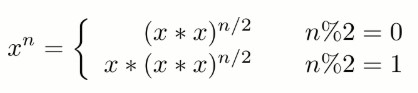• 数值的整数次方 java 题目描述 给定一个double类型的浮点数base和int类型的整数exponent。求base的exponent次方。 代码1： public class Solution { public double Power(double base, int exponent) { double a...
数值的整数次方 java
题目描述
给定一个double类型的浮点数base和int类型的整数exponent。求base的exponent次方。

代码1：

public class Solution {
public double Power(double base, int exponent) {
double a = 1.0;
if(exponent == 0){
a = 1;
}
if(exponent > 0){
for(int i = 0; i < exponent; i++){
a = a * base;
}
}
if(exponent < 0){
exponent = - exponent;
for(int i = 0; i < exponent; i++){
a = a * base;
}
a = 1 / a;
}
return a;
}
}


代码2：推荐

public class Solution {
public double Power(double base, int exponent) {
double a = 1.0;
if(exponent == 0){
a = 1;
}
if(exponent > 0 || exponent < 0){
for(int i = 0; i < exponent || i < - exponent; i++){
a = a * base;
}
if(exponent < 0){
a = 1 / a;
}
}
return a;
}
}



展开全文• * 2 ^ 4 = 16 4D = 100B * res = power(2,2) -> power(2,1) -> 2 * | * res&1=1 * 2*2 * 4*4 */ public class 数值的整数次方 { public static double power(double num, int exp) { if (exp )//解决负数问题 ...
偶数 A(n) = A(n/2) *  A(n/2)奇数A(n) = A((n-1)/2) * A((n-1)/2)指数为0或小于1 怎么办？底数为0 时返回什么？* 2 ^ 4 = 16   4D = 100B* res = power(2,2) -> power(2,1) -> 2*                        |*                     res&1=1*                      2*2*         4*4*/public class 数值的整数次方 {public static double power(double num, int exp) {if (exp < 0)//解决负数问题return 1 / power(num, -exp);if (exp == 0)return 1;if (exp == 1)return num;double res = power(num, exp >> 1);res = res * res;if ((exp & 1) == 1)res = res * num;return res;}public static void main(String[] args) {System.out.println(power(2, 4));System.out.println(power(2, -2));}}用右移运算替代了除以2用位与运算符替代了求余运算符(%)来判断奇偶当需要对2的次幂进行求余时，可以是使用&运算符来代替a/b的除数b必必须为2的n次方使用位操作(&运算)代替求余操作%运算:a%b由于我们知道位运算比较高效，在某些情况下，当b为2的n次方时，有如下替换公式：a % b = a & (b-1)(b=2n)即：a % 2n = a & (2n-1)例如：14%8，取余数，相当于取出低位，而余数最大为7，14二进制为1110，8的二进制1000，8-1 = 7的二进制为0111，由于现在低位全为1，让其跟14做&运算，正好取出的是其低位上的余数。1110&0111=110即6=14%8；(此公式只适用b=2n，是因为可以保证b始终只有最高位为1，其他二进制位全部为0，减去1，之后，可以把高位1消除，其他位都为1，而与1做&运算，会保留原来的数。)参考
展开全文• 数值的整数次方 给定一个 double 类型的浮点数 base 和 int 类型的整数 exponent，求 base 的 exponent 次方。 package com.kangin.other; /** * * @author 26800 * 给定一个 double 类型的浮点数 base 和 int ...
数值的整数次方
给定一个 double 类型的浮点数 base 和 int 类型的整数 exponent，求 base 的 exponent 次方。
package com.kangin.other;
/**
*
* @author 26800
* 给定一个 double 类型的浮点数 base 和 int 类型的整数 exponent，求 base 的 exponent 次方。
*
*/
public class NumberCapital {

public double Power(double base,int exponent) {
if (exponent == 0) {
return 1;
}
if (exponent == 1) {
return base;
}
boolean isNegative = false;                //添加标识符，以便最后结果判断
if (exponent < 0) {
exponent = -expontent;
isNegative = true;
}
double pow = Power(base*base, exponent/2); //递归求次方
if (exponent % 2 != 0)                     //说明expontent为奇数，需再乘上base
pow = pow * base;
return isNegative ? 1 / pow : pow;         //若为负数，则变为-1次方
}

}

此题主要求x的n次方，需对n进行分析，想出解题思路展开全文算法
• 12、数值的整数次方 题目描述： 给定一个double类型的浮点数base和int类型的整数exponent。求base的exponent次方。保证base和exponent不同时为0 思路： 把整数次方拆解成连乘，按指数分三种情况（正、负、零）考虑。...
12、数值的整数次方
题目描述：
给定一个double类型的浮点数base和int类型的整数exponent。求base的exponent次方。保证base和exponent不同时为0
思路：
把整数次方拆解成连乘，按指数分三种情况（正、负、零）考虑。
注：不要遗漏指数<0和底数为0这种情况，抛出异常。
public class Solution {
public double Power(double base, int exponent) {
double result = 1d;
if(Math.abs(base)<=1e-15&&exponent<0){throw new RuntimeException("error!");}
if(exponent==0){return 1;}
for(int i=0;i<Math.abs(exponent);i++){
result *= base;
}
return exponent>0 ? result:(1/result);
}
}

优化
public class Solution {
public double Power(double base, int exponent) {
double result = 1;
int n;
if(exponent>0){
n = exponent;
}else if(exponent<0){
if(Math.abs(base)<=1e-15)//此处double类型是否为0判断是和某一个精度范围比较，这样更准确！！
throw new RuntimeException("error!");
n = -exponent;
}else{// n==0
return 1;// 0的0次方
}
while(n!=0){
if((n&1)==1)//按位与，相同为1相异为0，此处是表示指数为奇数
result*=base;
//指数每右移一位相当于除以2，即开方，此时底数翻倍，相当于平方
base*=base;// 翻倍，即平方
n>>=1;// 右移一位，相当于除以2
}
return exponent>=0 ? result:(1/result);
}
}

这里的思路是快速幂，当指数为奇数时乘以底数，之后每次移位指数除以2，底数平方，比如2^50=((((2*2^2)^2)^2)^2*2)^2，使用移位操作，该思路能够对时间复杂度优化，例如使用for循环时4次方运算需要3次，但是移位操作4次方运算只需2次就可完成，时间复杂度优化至logn。
使用移位操作更快是因为计算机只有加法运算，乘法运算的运算需要多次的进位和转换，所以位运算的效率要远远高于乘法运算。


展开全文• 给定一个double类型的浮点数base和int类型的整数exponent。求base的exponent次方。 这道题看似简单，其实BUG重重。要注意的问题： 　1 关于次幂的问题特殊的情况，比如次幂为负数，或者基数为0时等等复杂的情况 ...
• 给定一个double类型的浮点数base和int类型的整数exponent。求base的exponent次方。 public class Solution { public double Power(double base, int exponent) { return Math.pow(base,exponent); } }power pow
• public class Solution { public double Power(double base, int exponent) { // 当底数为0，指数为负数时，则抛出异常或者返回0.0 if (equal(base, 0) && exponent < 0) { return 0.0;...
• 数值的整数次方 https://leetcode-cn.com/problems/shu-zhi-de-zheng-shu-ci-fang-lcof/ 实现函数double Power(double base, int exponent)，求base的exponent次方。不得使用库函数，同时不需要考虑大数问题。 ...
• 题目描述给定一个double类型的浮点数base和int类型的整数exponent。求base的exponent次方。这道题看似简单，其实BUG重重。要注意的问题：1 关于次幂的问题特殊的情况，比如次幂为负数，或者基数为0时等等复杂的情况2...
• 给定一个double类型的浮点数base和int类型的整数exponent。求base的exponent次方。 保证base和exponent不同时为0 示例1 输入 2,3 返回值 8.00000 二、代码 public class Solution { public double Power(double...
• 实现函数double Power(double base, int exponent)，求baseexponent次方。不得使用库函数，同时不需要考虑大数问题。 示例 1: 输入: 2.00000, 10 输出: 1024.00000 来源：力扣（LeetCode） 链接：...
• 数值的整数次方 实现函数double Power(double base, int exponent)，求base的exponent次方。不得使用库函数，同时不需要考虑大数问题。 示例 1: 输入: 2.00000, 10 输出: 1024.00000 示例 2: 输入: 2.10000, 3 输出...
• 数值的整数次方 解题思路 最直观的解法是将 x 重复乘 n 次，即 x*x*x…*x，时间复杂度为 O(N)。因为乘法是可交换的，所以当 n 为偶数时，可以将上述操作拆开成两半 (x*x…*x) * (x*x…*x)，两半的计算是一样的，...leetcode 算法
• public class 数值的整数次方 { public static double power(double num, int exp) { if (exp )//解决负数问题 return 1 / power(num, -exp); if (exp == 0) return 1; if (exp == 1) return num; double ...
• 给定一个double类型的浮点数base和int类型的整数exponent。求base的exponent次方。 保证base和exponent不同时为0 题解: 快速幂 1.如果base 等于0，则返回0. 2.如果exponent < 0，让 base = 1 / basleetcode
• 给定一个double类型的浮点数base和int类型的整数exponent。求base的exponent次方。 思路：首先先考虑特殊输入，考虑特殊的输入值，这里把0的负数次方定义为返回值为0，把0的0次方定位为1。为了保证效率，这里采用 ...剑指offer
• 给定一个double类型的浮点数base和int类型的整数exponent。求base的exponent次方。 2.算法描述 如果直接累乘时间复杂度是O(n)O(n)O(n)，调用库函数即可。 讨论下快速幂（时间复杂度为O(logn)O(logn)O(logn)）是...
• 代码 class Solution { public double myPow(double x, int n) { if(x==0) return 0; if(n==0) return 1; // n=−2147483648取-n，超出了int范围，所以先用long存放 long b=n; if(b<... }leetcode 算法
• 题目：数值的整数次方  实现函数 double Power(double base,int exponent),求base的exponent次方。不得使用库函数，同时不需要考虑大数问题。 算法分析： 我们都知道在C语言库函数中有一个pow函数...剑指offer 算法 面试题
• 实现函数double power(double base,int exponent)，求baseexponent次方。不得使用库函数，同时不需要考虑大数问题。 public class Power { public static void main(String args[]) { System.out.println...
• 1.当指数大于等于0时候，底数可以取任意double类型值，这里暂不考虑00次方是1还是0。 2.当指数小于0时： （1）如果底数为0，则在取倒数时候会出现分母为0情况，这是计算机不允许，这里让函数返回0.0，...面试题 算法 剑指offer
• 书中方法：这道题要注意底数为0情况。double类型相等判断。乘方递归算法。 public double power(double base, int exponent){ //指数为0 if(exponent == 0){ return 1.0; } //底数为0 i...
• 题目：实现函数double power( double base, int exponent)，求baseexponent次方。不得使用库函数，同时需要考虑大数问题。 大体思路很简单啊，就是base做exponent次相乘就好了。但是我们需要全面，比如base为0，...
• 使用快速幂的迭代来实现，... * 数值的整数次方：不可能是循环n次，因为会超时的。 * 快速幂 * 快速幂的迭代写法 */ public class shuZhiZhengShuCiFang { public static void main(String[] args) { double x =.
• 数值的整数次方Java） 题目：给定一个double类型的浮点数base和int类型的整数exponent。求base的exponent次方 思路： ① 指数的正负，我们可以先用绝对值，然后求出指数次方之后的结果，如果是负数取倒数即可； ②...算法 数据结构 leetcode...

# 数值的整数次方javajava 订阅